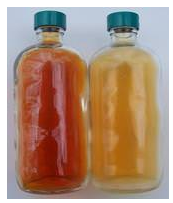# 3.1: Molecular Compounds

•• Contributed by Allison Soult
• Senior Lecturer (Chemistry) at University of Kentucky

Learning Outcomes

• Describe types of elements that form covalent bonds.
• Explain how a covalent bond is formed.
• Distinguish between ionic and molecular compounds.
• Know the meaning of prefixes used in naming molecular compounds.
• Distinguish between molecules and compounds.

Water and diamonds - two very different materials. Water can be found almost everywhere. It is in lakes, creeks, rivers, and oceans. We get water from the sky when it rains. Diamonds, on the other hand, are very rare. They are only found in a few locations on the earth and must be mined to become available. Major diamond mines are located in various African countries, Australia, and Russia. The United States has several underground sources of diamonds in Alaska, Colorado, Minnesota, Montana, and Wyoming, but the only "active" U.S. mine is the Crater of Diamonds mine in a state park near Murfreesboro, Arkansas. For a small fee, visitors can dig for diamonds. You won't get rich by visiting, though - only a few hundred carats of low-grade diamonds are found each year.

The two materials do have at least one thing in common. The atoms in the materials are held together by covalent bonds. These bonds consist of electrons shared between two or more atoms. Unlike ionic bonds, where electrons are either lost or gained by an atom to form charged ions, electrons in covalent compounds are shared between the two atoms, giving rise to properties that are quite different from those seen in ionic materials.

## Molecular Compounds

Molecular compounds are chemical compounds that take the form of discrete molecules. Examples include such familiar substances as water $$\left( \ce{H_2O} \right)$$ and carbon dioxide $$\left( \ce{CO_2} \right)$$ (Figure $$\PageIndex{1}$$). These compounds are very different from ionic compounds like sodium chloride $$\left( \ce{NaCl} \right)$$. Ionic compounds are formed when metal atoms lose one or more of their electrons to nonmetal atoms. The resulting cations and anions are electrostatically attracted to each other.

So what holds the atoms of a molecule together? Rather than forming ions, the atoms of a molecule share their valence electrons in such a way that a bond forms between pairs of atoms. In a carbon dioxide molecule, there are two of these bonds, each occurring between the carbon atom and one of the two oxygen atoms.Figure $$\PageIndex{1}$$: Carbon dioxide molecules consist of a central carbon atom bonded to two oxygen atoms.

## Binary Molecular Compound Names

Recall that a molecular formula shows the number of atoms of each element that a molecule contains. A molecule of water contains two hydrogen atoms and one oxygen atom, so its formula is $$\ce{H_2O}$$. A molecule of octane, which is a component of gasoline, contains eight atoms of carbon and eighteen atoms of hydrogen. The molecular formula of octane is $$\ce{C_8H_{18}}$$.

A binary molecular compound is a molecular compound that is composed of two elements. In general, the elements that combine to form binary molecular compounds are both nonmetals. This contrasts with ionic compounds, which involve bonds between metal cations and nonmetal anions. One difference between ionic and molecular compounds is that two nonmetal atoms will frequently combine with one another in a variety of ratios. Consider the elements nitrogen and oxygen. They combine to make several binary compounds, including $$\ce{NO}$$, $$\ce{NO_2}$$, (see figure below), and $$\ce{N_2O}$$. Obviously they can't all be called nitrogen oxide! How would someone know which one you were talking about? Each of the three compounds has very different properties and reactivity. A system to distinguish between compounds such as these is necessary.Figure $$\PageIndex{2}$$: $$\ce{NO_2}$$ (left) is a reddish-brown toxic gas that is a prominent air pollutant produced by internal combustion engines. It is converted to $$\ce{N_2O}$$ (right) at low temperatures.

Prefixes are used in the names of binary molecular compounds to identify the number of atoms of each element. Although we will not cover nomenclature rules for molecular compounds you should be familiar with the prefixes used in their names. Listed below (see table below) are the prefixes up to ten.

Number of Atoms Prefix
Table $$\PageIndex{1}$$: Numerical Prefixes
1 mono-
2 di-
3 tri-
4 tetra-
5 penta-
6 hexa-
7 hepta-
8 octa-
9 nona-
10 deca-

Some examples of molecular compounds are listed below (see table below). Note the use of prefixes in their names.

Formula Name
Table $$\PageIndex{2}$$: Examples of Molecular Compounds
$$\ce{NO}$$ nitrogen monoxide
$$\ce{N_2O}$$ dinitrogen monoxide
$$\ce{S_2Cl_2}$$ disulfur dichloride
$$\ce{Cl_2O_7}$$ dichlorine heptoxide